• 主要介绍了matlab画三维图像的示例代码(附demo),文中通过示例代码介绍的非常详细，对大家的学习或者工作具有一定的参考学习价值，需要的朋友们下面随着小编来一起学习学习吧
• 主要介绍了Python实现读取txt文件并画三维图简单代码示例，具有一定借鉴价值，需要的朋友可以参考下。
• 该例程实现了VS2010下利用TeeChart控件绘制三维图
• ## matlab 画三维图像

千次阅读 2018-08-14 11:11:43
数学学习中，有很多地方需要画图来直观对比显示，本节记录一下用matlab画三维图形的几种方法。 例：, 1、surf、surfc、surfl surf:   clear clc close all %% gride [x,y]=meshgrid(0:0.1:3,0:0.1:3); %% ...
数学学习中，有很多地方需要画图来直观对比显示，本节记录一下用matlab画三维图形的几种方法。
例：,
1、surf、surfc、surfl

surf:

clear
clc
close all
%% gride
[x,y]=meshgrid(0:0.1:3,0:0.1:3);

%% plot
z = x.^2-y;
surf(x,y,z)
xlabel('X')
ylabel('Y')
zlabel('Z')
title('z=x^2-y')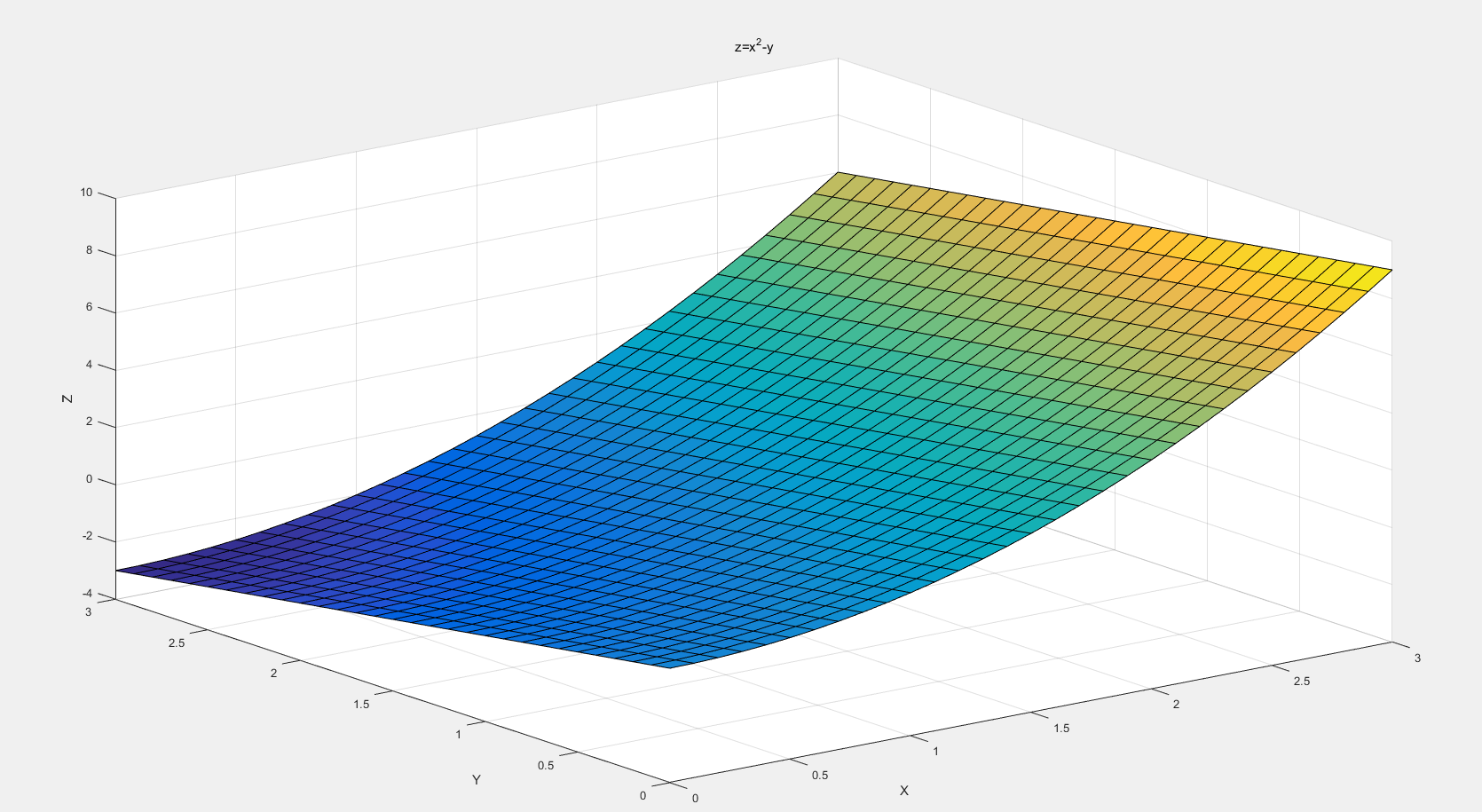surfc：有等高线
surfc(x,y,z)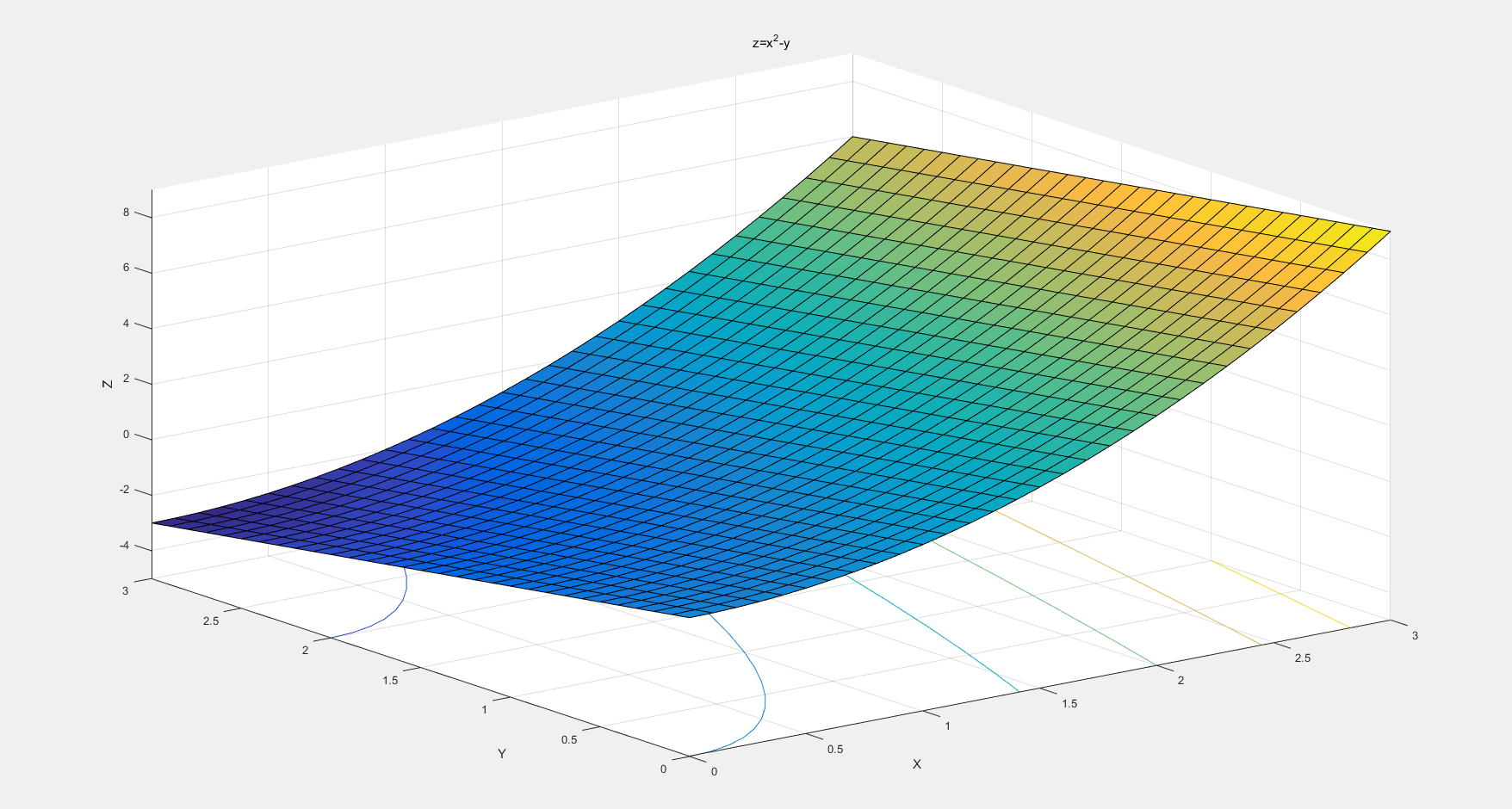surfl：MATLAB中内置了surfl函数，基于运用漫反射、镜面发射光和环境照明模型。使用一个单色颜色映像（如灰色，纯白，铜黄或粉红色）和插值色彩，会画出效果更好的曲面
surfl(x,y,z,s)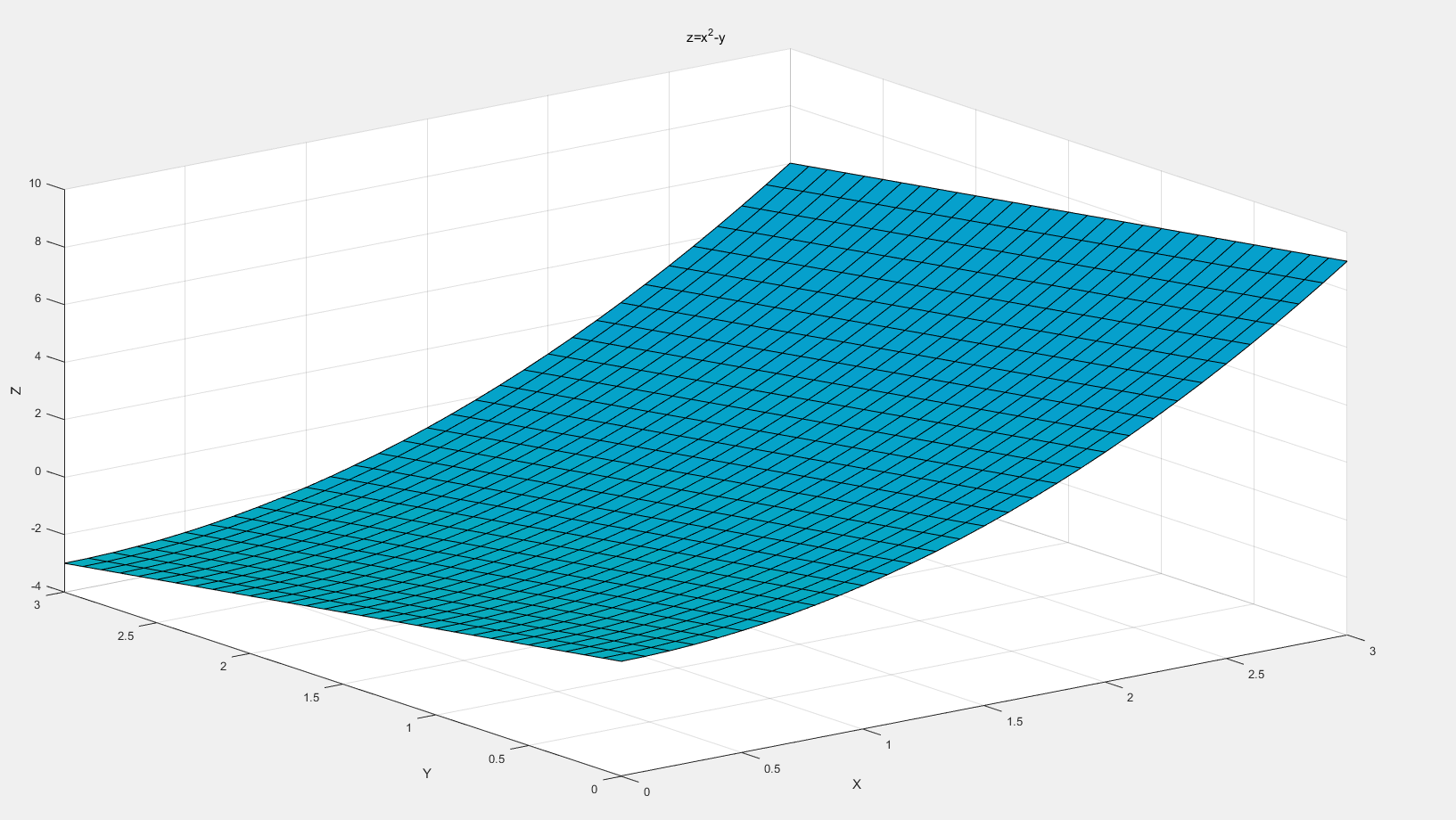2、mesh
clear
clc
close all
%% grid
[x,y]=meshgrid(0:0.1:3,0:0.1:3);

%% first
% plot
z = x.^2-y;
c=zeros(31,31);
c=c+0.1;
mesh(x,y,z,c)
xlabel('X')
ylabel('Y')
zlabel('Z')
% make label
hold on
s='x^2-y';
text(x(1,31),y(1,31),z(1,31),s,'fontsize',15,'HorizontalAlignment','center');

%% second
% plot
hold on
z1=sqrt(x)-y;
c1=c+0.2;
mesh(x,y,z1,c1)
% make label
hold on
s='√x-y';
text(x(1,31),y(1,31),z1(1,31),s,'fontsize',15,'HorizontalAlignment','center');

%% third
% plot
hold on
z2=sqrt(x+y)-2*x;
c2=c+0.3;
mesh(x,y,z2,c2)
% make lable
hold on
s='√(x+y)-2y';
text(x(1,31),y(1,31),z2(1,31),s,'fontsize',15,'HorizontalAlignment','center');

title('x^2-y,√x-y,√(x+y)-2y')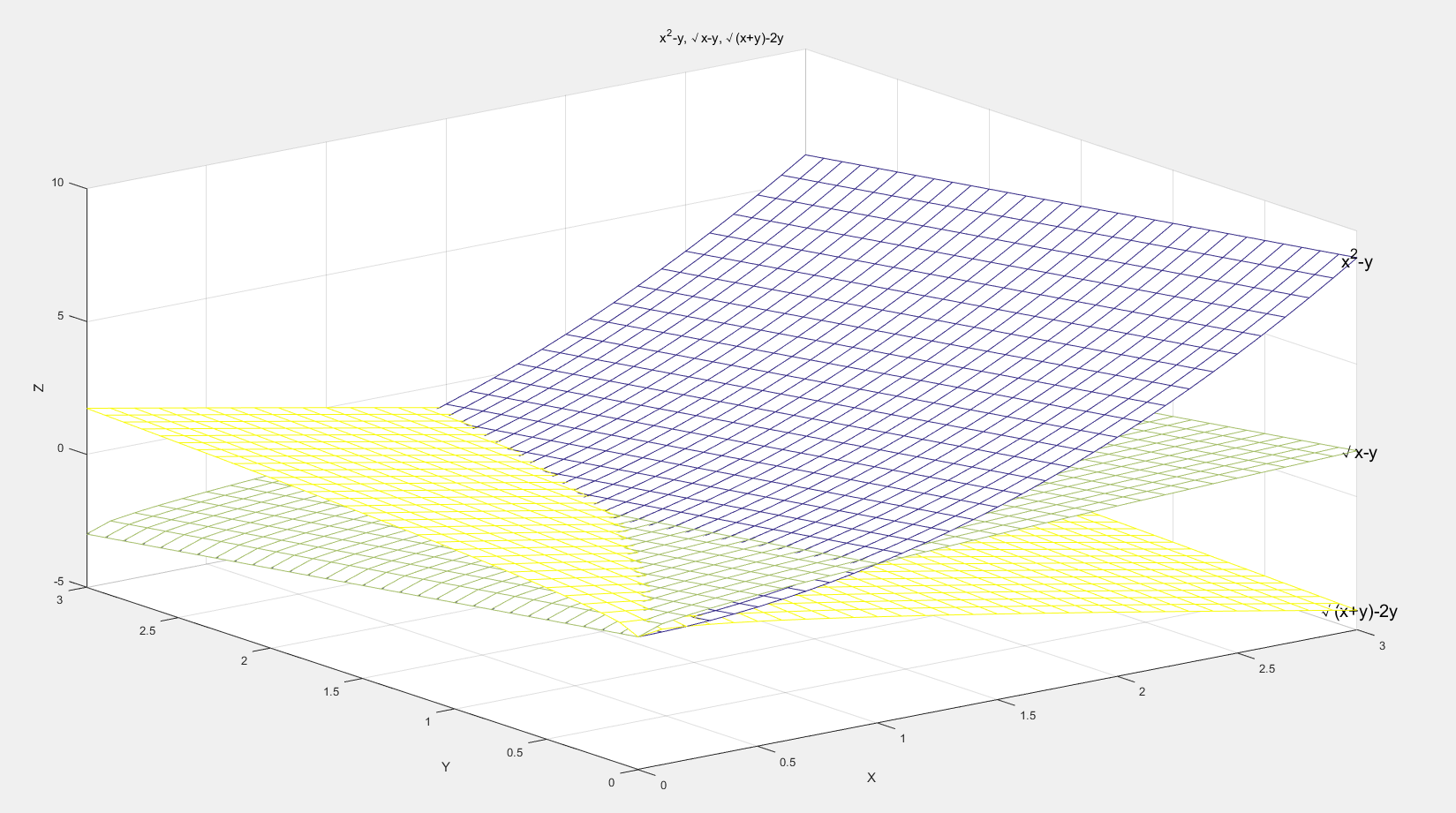展开全文• 今天小编就为大家分享一篇python 画三维图像 曲面和散点的示例，具有很好的参考价值，希望对大家有所帮助。一起跟随小编过来看看吧
• ## Python画三维图

千次阅读 多人点赞 2020-07-24 20:06:02
from mpl_toolkits.mplot3d import Axes3D import matplotlib.pyplot as plt import numpy as np import pandas as pd my_dpi=96 plt.figure(figsize=(480/my_dpi, 480/my_dpi), dpi=my_dpi) ...
from mpl_toolkits.mplot3d import Axes3D
import matplotlib.pyplot as plt
import numpy as np
import pandas as pd

my_dpi=96
plt.figure(figsize=(480/my_dpi, 480/my_dpi), dpi=my_dpi)

df=pd.DataFrame({'X': range(1,101), 'Y': np.random.randn(100)*15+range(1,101), 'Z': (np.random.randn(100)*15+range(1,101))*2 })

fig = plt.figure()
ax.scatter(df['X'], df['Y'], df['Z'], c='skyblue', s=60)
ax.view_init(30, 185)
plt.show()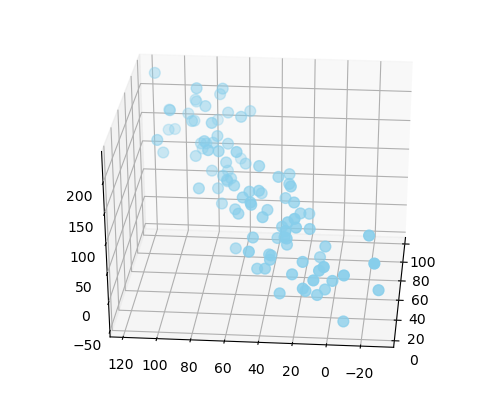from mpl_toolkits.mplot3d import Axes3D
import matplotlib.pyplot as plt
import pandas as pd
import seaborn as sns

my_dpi=96
plt.figure(figsize=(480/my_dpi, 480/my_dpi), dpi=my_dpi)

df=data.unstack().reset_index()
df.columns=["X","Y","Z"]

df['X']=pd.Categorical(df['X'])
df['X']=df['X'].cat.codes

fig = plt.figure()
ax = fig.gca(projection='3d')
surf=ax.plot_trisurf(df['Y'], df['X'], df['Z'], cmap=plt.cm.viridis, linewidth=0.2)
fig.colorbar( surf, shrink=0.5, aspect=5)
plt.show()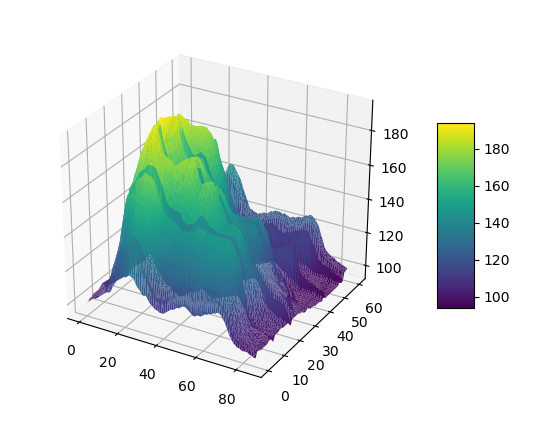from mpl_toolkits.mplot3d import Axes3D
import matplotlib.pyplot as plt
import pandas as pd
import seaborn as sns

my_dpi=96
plt.figure(figsize=(480/my_dpi, 480/my_dpi), dpi=my_dpi)

df=data.unstack().reset_index()
df.columns=["X","Y","Z"]

df['X']=pd.Categorical(df['X'])
df['X']=df['X'].cat.codes

fig = plt.figure()
ax = fig.gca(projection='3d')
ax.plot_trisurf(df['Y'], df['X'], df['Z'], cmap=plt.cm.jet, linewidth=0.01)
plt.show()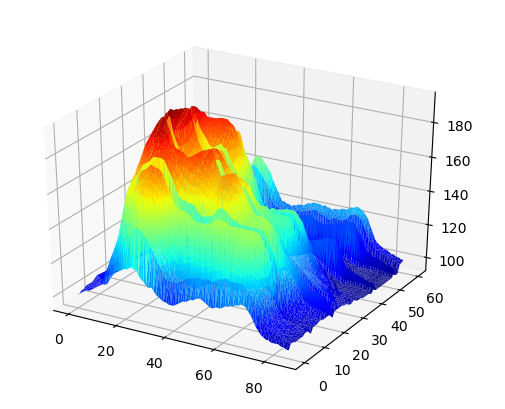import pandas as pd
import numpy as np
from sklearn.decomposition import PCA
import matplotlib.pyplot as plt

import seaborn as sns
sns.set_style("white")

my_dpi=96
plt.figure(figsize=(480/my_dpi, 480/my_dpi), dpi=my_dpi)

df['species']=pd.Categorical(df['species'])
my_color=df['species'].cat.codes
df = df.drop('species', 1)

pca = PCA(n_components=3)
pca.fit(df)

result=pd.DataFrame(pca.transform(df), columns=['PCA%i' % i for i in range(3)], index=df.index)

fig = plt.figure()
ax.scatter(result['PCA0'], result['PCA1'], result['PCA2'], c=my_color, cmap="Set2_r", s=60)

xAxisLine = ((min(result['PCA0']), max(result['PCA0'])), (0, 0), (0,0))
ax.plot(xAxisLine, xAxisLine, xAxisLine, 'r')
yAxisLine = ((0, 0), (min(result['PCA1']), max(result['PCA1'])), (0,0))
ax.plot(yAxisLine, yAxisLine, yAxisLine, 'r')
zAxisLine = ((0, 0), (0,0), (min(result['PCA2']), max(result['PCA2'])))
ax.plot(zAxisLine, zAxisLine, zAxisLine, 'r')

ax.set_xlabel("PC1")
ax.set_ylabel("PC2")
ax.set_zlabel("PC3")
ax.set_title("PCA on the iris data set")
plt.show()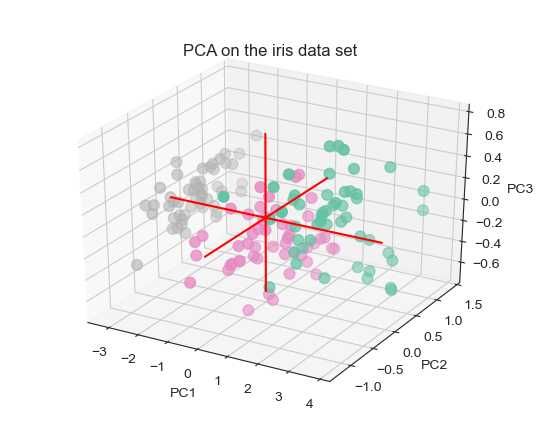import matplotlib.pyplot as plt
import pandas as pd
from math import pi

df = pd.DataFrame({
'group': ['A','B','C','D'],
'var1': [38, 1.5, 30, 4],
'var2': [29, 10, 9, 34],
'var3': [8, 39, 23, 24],
'var4': [7, 31, 33, 14],
'var5': [28, 15, 32, 14]
})

def make_spider( row, title, color):

categories=list(df)[1:]
N = len(categories)

angles = [n / float(N) * 2 * pi for n in range(N)]
angles += angles[:1]

ax = plt.subplot(2,2,row+1, polar=True, )

ax.set_theta_offset(pi / 2)
ax.set_theta_direction(-1)

plt.xticks(angles[:-1], categories, color='grey', size=8)

ax.set_rlabel_position(0)
plt.yticks([10,20,30], ["10","20","30"], color="grey", size=7)
plt.ylim(0,40)

values=df.loc[row].drop('group').values.flatten().tolist()
values += values[:1]
ax.plot(angles, values, color=color, linewidth=2, linestyle='solid')
ax.fill(angles, values, color=color, alpha=0.4)

# 添加标题
plt.title(title, size=11, color=color, y=1.1)

my_dpi=96
plt.figure(figsize=(1000/my_dpi, 1000/my_dpi), dpi=my_dpi)

my_palette = plt.cm.get_cmap("Set2", len(df.index))

for row in range(0, len(df.index)):
make_spider( row=row, title='group '+df['group'][row], color=my_palette(row))
plt.show()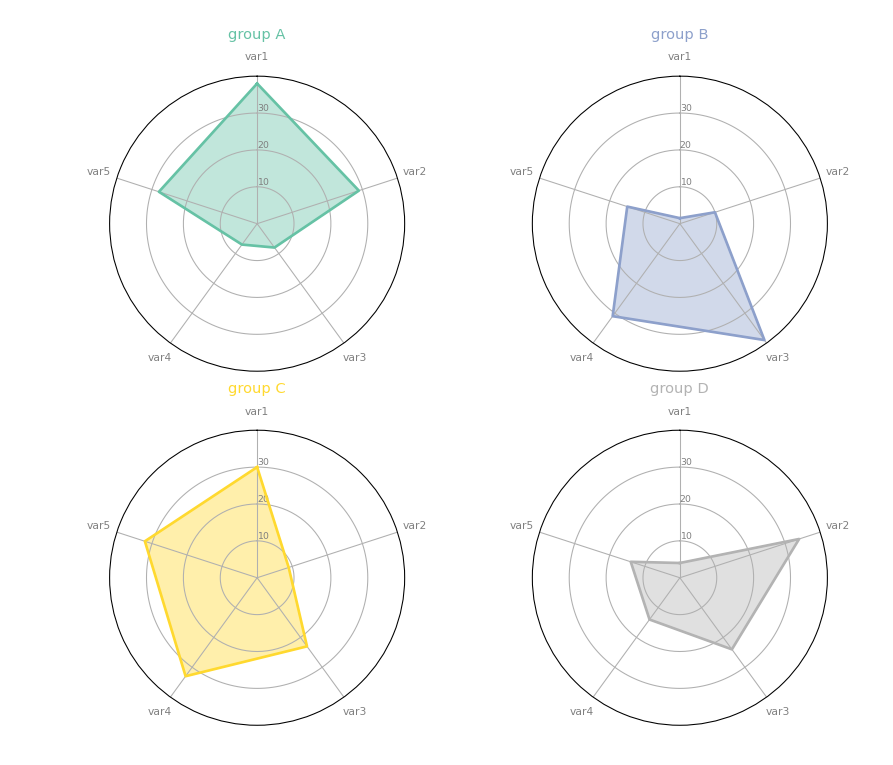本博主新开公众号， 希望大家能扫码关注一下，十分感谢大家。本文来自：https://github.com/holtzy/The-Python-Graph-Gallery/blob/master/PGG_notebook.py 
展开全文python
• opengl画三维图像，该实例包括MFC 下opengl环境的搭建，三维坐标的简历，画三维图像。是完整的实例，能运行。opengl MFC
• ## Matlab画三维图的一些技巧

千次阅读 多人点赞 2020-05-17 18:04:00
Matlab画三维图 Matlab画三维图形的需求通常是针对二元函数而言的，Matlab画图主要采用描点的方法，就是画出给定点对应的值，然后Matlab会自动把这些点连接起来，就成了很漂亮的一幅图。所以你给的点越多，画的图就...
引言
本人是一位数学科研工作者，平时的文章采用的是latex编写，里面图形的生成主要来自于Matlab（个人对Matlab非常喜欢，感觉上手比较容易，更亲民）。对于图形的处理比较频繁，而且总会有一些特殊的需求，每次都要上网搜查，或者查以前用过的命令，经常花了很多时间，实现了一点小要求，事后回想有点得不偿失。因此借助这个平台，记录自己在Matlab使用过程中碰到的一些问题，给出我找到或者知道的解决办法，不过方法不是唯一的，也希望广大网友能有更好的思路提供。后续碰到的问题我也会继续更新（如果我有时间的话哈）。
Matlab画三维图
Matlab画三维图形的需求通常是针对二元函数而言的，Matlab画图主要采用描点的方法，就是画出给定点对应的值，然后Matlab会自动把这些点连接起来，就成了很漂亮的一幅图。所以你给的点越多，画的图就越精确。
一个简单的例子
下面我们以函数

u

=

s

i

n

(

x

)

s

i

n

(

y

)

u=sin(x)sin(y)

为例，画三维图形的代码如下：
function PF_3D
px=100; py=150; %x轴我布100个点，y方向布150个点
x=linspace(0,2*pi,px); %该函数表示在[0,2*pi]上均匀布px个点
y=linspace(0,2*pi,py);
[X,Y]=meshgrid(x,y); %该函数的作用详见下文
u=sin(X).*sin(Y); %计算对应点的函数值u %注意点乘
surf(X,Y,u); %画图
%mesh(X,Y,u);
end

代码中的meshgrid命令是生成

m

×

n

m\times n

的X,Y两个矩阵，这里m是y的长度，n是x的长度，结果如下图Matlab代码X就是把x向量复制了m行，每行都一样。Y就是把y复制了n列，每列都一样，这样X，Y矩阵相同位置的值就对应了点的横纵坐标（总共有

m

×

n

m\times n

个点），再由前面代码计算得到u就得到点对应的函数值。最后可以通过mesh或者surf命令进行画图就。mesh和surf是有区别的，但是我也没去区分，我自己常用的是surf命令，下面看两个命令画出图的不同(左边是surf，右边是mesh)上图中左边surf画出来的图比较黑那是因为Matlab在图上画了黑色网格线，如果要去掉他可以在后面加命令：
shading interp

效果如图：如果不喜欢后面坐标平面的那些网格线，也可以加命令：
grid off

就可以让它消失。
坐标轴的设置
1.从上面的图可以看出，如果不加干预的话，Matlab画出你给的横纵数值范围的全部图形，但有些时候我们可能比较关心局部的图样，这时候就要设置图形坐标轴的显示范围，只要在surf命令后面加命令：
axis([0,2,0,4,0,1])

axis里面参数分别对应x_min,x_max,y_min,y_max,z_min,z_max,效果如图2.有些问题他的图形是长条形的，如x轴应该很长，y，z轴比较短的，但Matlab总是画得“方方正正”，这样就会变得不美观（肯定有个轴被压缩），所以此时我们选择控制轴的相对长度，可以在surf后面加命令：
pbaspect([2，1，1]);

pbaspect后面得三个参数表示横纵竖得长度比例是2：1：1，最后效果如图：（我们这个例子不需要让轴变长，拉长反而不好看，为了演示拼了）3.隐藏坐标轴。有时候我们保存多张图，只是为了体现图形的变化，这样就不需要每个坐标轴刻度和数值都显示出来，所以我们要隐藏坐标轴，这个在网上有很多人提供了方案，但是个人觉得比较好用的方案是在surf后面添加如下命令
 t=0:0:0;
set(gca,'xtick',t); %这两句话可以去掉x轴的刻度和坐标值
xlabel('x轴的说明') %这句话可以坐标的下面添加一个说明
set(gca,'ytick',t); %这两句话可以去掉y轴的刻度和坐标值
ylabel('y轴的说明') %这句话可以坐标的下面添加一个说明
set(gca,'ztick',t); %这两句话可以去掉y轴的刻度和坐标值
zlabel('z轴的说明') %这句话可以坐标的下面添加一个说明

直接看效果横纵竖的刻度和刻度值都消失了，留下了三条边缘黑线，至于这个怎么去了目前还不知道（是不是可以设置刻度线的颜色成白色就行？）。上面的方案原出处可以在该网页查看。 4.其他坐标轴设置。还有很多坐标轴的设置可以直接上网查找，或者直接编辑图片，如何编辑呢？就是直接在生成的图像窗口点查看-属性编辑器（我用的2019版本的是这样，在以前的版本在图形窗口有个图标直接点就行了，但是19版本没有了，感觉没有了很难受，谁知道怎么添加这个属性编辑器的快捷图标到工具栏里）。进入属性编辑器之后，就会发现里面有好多对图形的坐标轴的设置，还是挺好用的，但是如果对很多图同时处理的画，这样效率很低，还是能用代码解决的就用代码解决。
图形的视角
有时候我们不需要三维的立体图，可能只要俯视图或者侧视图，通过图形的颜色区别函数值的大小。这时候我们就需要对图形进行旋转，一种办法就是直接对生成图形进行旋转(看工具栏上有，或者鼠标移到图上就有这个旋转工具跳出来)。第二种办法就是加命令
view(0,90) %俯视图xoy平面，x为横轴
%view(-90,0) %侧视图yoz平面，y为横轴
%view(0,0) %侧视图xoz平面，x为横轴

效果如图但这样光有颜色没有标明颜色对应的值，我们可以在图形窗口点插入颜色栏图标（上图工具栏中的第六个图标）会在右侧出现颜色栏。如果对这个颜色栏不喜欢，可以右击刚生成的颜色栏选择标准颜色图，选自己喜欢的打勾就行（我比较喜欢jet）。上面的过程也可以直接采用如下命令实现：
colorbar  %显示颜色栏
colormap(jet)  %颜色的风格选择

效果如下：图像的保存
图像的保存一种就是直接在图形窗口，文件-另存为就有好多的格式供你选择，我们写论文的时候一般要求是eps格式的图片，因为它是矢量图，放大不失真，Matlab有提供这个选择，两个字完美！这种通过选择保存图片的方式非常笨拙，一两个图还行，多个图的产生，或者程序需要长时间运行的不想干等的就比较麻烦，所以还是代码来解决吧。
我们以一个例子来爽快的解决这个问题。比如我们打算画函数图

u

=

s

i

n

(

k

x

)

c

o

s

(

k

y

)

u=sin(kx)cos(ky)

这里的

k

k

的取值[1,2,3],现在要将每个

k

k

对应的图保存到当前路径的figures文件夹里（这个文件夹要原先要存在），文件命保存成fg1.fig，fg2.fig，fg3.fig（.fig是Matlab图形保存是默认的后缀名，这样后缀的图形可以再由Matlab打开）。给出如下代码：
function PFS
px=100; py=150; %x轴我布100个点，y方向布150个点
x=linspace(0,2*pi,px); %该函数表示在[0,2*pi]上均匀布px个点
y=linspace(0,2*pi,py);
[X,Y]=meshgrid(x,y); %该函数的作用详见下文
fid=[pwd,'\figures\']; %这个命令用fid指当前路径下的figures文件夹
for k=1:3
u=sin(k*X).*sin(k*Y); %计算对应点的函数值u %注意点乘
figure(k) %创建第k个图形窗口
surf(X,Y,u); %画图
M=int2str(k);%将k转化成字符存到M中
saveas(gcf,[fid,'f',M,'.fig']);
end
end

上段代码的核心：1.创建图窗 2.保存图形。先看创建图窗的命令就是
figure(k) %创建第k个图形窗口

注意k一定要加括号，没有括号不行。这个figure命令是k的for循环命令内，这样就可以随着k的变化创建不同的图窗，然后再fiugure(k)后面跟上画图命令，图就会画到相应的图窗。这样就避免了后面画的图覆盖前面画的图情形。接着就是保存图形，我们首先再for循环的外围给命令
fid=[pwd,'\figures\'];

这个fid变量是为了保存图像要保存的路径，参数pwd是Matlab自带的表示当前路径，\figures\就是figures文件夹（因为所有的图都要保存到这个文件夹里，所以这个命令放在for循环的外面）。在for循环内容我们要给图像名字起fg1.fig，fg2.fig，fg3.fig，注意名字上的1，2，3其实就是k的值，但是k是个数值（整数值），而文件名应该是个字符串，所以首先先将k转化成字符串
 M=int2str(k);%将k转化成字符存到M中

然后再合成一个完整的路径就是[fid,‘f’,M,’.fig’]，这个中括号，中间逗号起到了字符串左右拼接的作用，注意里面已经是字符串了不用加引号，不是的要加。最后通过saveas函数进行图像保存：
saveas(gcf,[fid,'f',M,'.fig']);

saveas第一个参数gcf我也不知什么意思，是否是获取图片的意思，我就当默认处理了。 如果是要将图片保存成eps格式的画，要把saveas的参数命令中的’.fig’改成’.eps’，但如果仅仅如此的话，保存最终的eps图像是黑白的（我的Matlab是这样，不知道你们是否也是），如果想要是彩色的，要在后面额外加一个参数’psc2’，具体代码如下：
saveas(gcf,[fid,'f',M,'.eps'],'psc2');

这样就大功告成。
结束语
如果读者有碰到一些Matlab的问题也可以留言提问，如果我知道的话也会告知。
文中很多方法是我看书或者搜索到的，如果有侵权请告知，我深表歉意。
展开全文matlab
• 最基本的三维图是由(x, y, z)三维坐标点构成的线图与散点图，可以用ax.plot3D和ax.scatter3D函数来创建，默认情况下，散点会自动改变透明度，以在平面上呈现出立体感三维的线图和散点图...toolkitsimport mplot3d%...
最基本的三维图是由(x, y, z)三维坐标点构成的线图与散点图，可以用ax.plot3D和ax.scatter3D函数来创建，默认情况下，散点会自动改变透明度，以在平面上呈现出立体感
三维的线图和散点图
1
2
3
4
5
6
7
8
9
10
11
12
13
14
15
16
17
18
19
#绘制三角螺旋线
from mpl_toolkitsimport mplot3d
%matplotlib inline
import matplotlib.pyplot as plt
import numpy as np
ax= plt.axes(projection='3d')
#三维线的数据
zline= np.linspace(0,15,1000)
xline= np.sin(zline)
yline= np.cos(zline)
ax.plot3D(xline, yline, zline,'gray')
# 三维散点的数据
zdata= 15 * np.random.random(100)
xdata= np.sin(zdata)+ 0.1 * np.random.randn(100)
ydata= np.cos(zdata)+ 0.1 * np.random.randn(100)
ax.scatter3D(xdata, ydata, zdata, c=zdata, cmap='Greens')三维等高线图
1
2
3
4
5
6
7
8
9
10
11
12
13
14
15
def f(x, y):
return np.sin(np.sqrt(x** 2 + y** 2))
x= np.linspace(-6,6,30)
y= np.linspace(-6,6,30)
X, Y= np.meshgrid(x, y)
Z= f(X,Y)
fig= plt.figure()
ax= plt.axes(projection='3d')
ax.contour3D(X, Y, Z,50, cmap='binary')
ax.set_xlabel('x')
ax.set_ylabel('y')
ax.set_zlabel('z')
#调整观察角度和方位角。这里将俯仰角设为60度，把方位角调整为35度
ax.view_init(60,35)线框图和全面图
全面图和线框图相似，只不过线框图的每一个面都是由多边形构成。只要增加唉一个配色方案来填充这些多边形，就可以感受到可视化图形表面的拓扑结构了。
1
2
3
4
5
#线框图
fig=plt.figure()
ax= plt.axes(projection='3d')
ax.plot_wireframe(X, Y, Z, color='c')
ax.set_title('wireframe')1
2
3
4
#曲面图
ax= plt.axes(projection='3d')
ax.plot_surface(X, Y, Z, rstride=1, cstride=1, cmap='viridis', edgecolor='none')
ax.set_title('surface')1
2
3
4
5
6
7
8
9
#使用极坐标可以获得切片的效果
r= np.linspace(0,6,20)
theta= np.linspace(-0.9 * np.pi,0.8 * np.pi,40)
r, theta= np.meshgrid(r, theta)
X= r* np.sin(theta)
Y= r* np.cos(theta)
Z= f(X, Y)
ax= plt.axes(projection='3d')
ax.plot_surface(X, Y, Z, rstride=1, cstride=1, cmap='viridis', edgecolor='none')曲面三角剖分
在某些应用场景下，上述这些要求均匀采样的网格数据显得太过严格且不太容易实现。这时就可以使用三角剖分部分图形。
1
2
3
4
5
6
7
8
theta= 2 * np.pi* np.random.random(1000)
r= 6 * np.random.random(1000)
x= np.ravel(r* np.sin(theta))
y= np.ravel(r* np.cos(theta))
z= f(x, y)
ax= plt.axes(projection='3d')
ax.scatter(x, y, z, c=z, cmap='viridis', linewidth=0.5)1
2
3
#上图还有许多地方需要修补，这些工作可以由ax.plot_trisurf函数帮助我们完成。它首先找到一组所有点都连接起来的三角形，然后用这些三角形创建曲面
ax= plt.axes(projection='3d')
ax.plot_trisurf(x, y, z, cmap='viridis', edgecolor='none')莫比乌斯带（应用曲面三角剖分）
1
2
3
4
5
6
7
8
9
10
11
12
13
14
15
16
17
18
19
20
#绘制莫比乌斯带
#由于它是一条二维带，因此需要两个内在维度。theta维度取值范围是0～2pi，宽度维度w取值范围是-1～1
theta= np.linspace(0,2 * np.pi,30)
w= np.linspace(-0.25,0.25,8)
w, theta= np.meshgrid(w, theta)
phi= 0.5 * theta
#x-y平面内的半径
r= 1 + w* np.cos(phi)
x= np.ravel(r* np.cos(theta))
y= np.ravel(r* np.sin(theta))
z= np.ravel(w* np.sin(phi))
#要画出莫比乌斯带，还必须保证三角部分是正确的。最好的方法是首先用基本参数化方法定义三角部分，然后用Matplotlib将
#这个三角剖分映射到莫比乌斯带的三维空间里
from matplotlib.triimport Triangulation
tri= Triangulation(np.ravel(w), np.ravel(theta))
ax= plt.axes(projection='3d')
ax.plot_trisurf(x, y, z, triangles=tri.triangles, cmap='viridis', linewidth=0.2)
ax.set_xlim(-1,1);ax.set_ylim(-1,1);ax.set_zlim(-1,1)到此这篇关于如何用Matplotlib 画三维图的示例代码的文章就介绍到这了,更多相关Matplotlib 三维图内容请搜索服务器之家以前的文章或继续浏览下面的相关文章希望大家以后多多支持服务器之家！
原文链接：https://blog.csdn.net/jasonzhoujx/article/details/81780774
展开全文• 使用 matlab 绘制三维图形的方法 三维曲线 plot3 函数与 plot 函数用法十分相似其调用格式为 plot3(x1,y1,z1,选项 1,x2,y2,z2, 选项 2,xn,yn,zn,选项 n)其中每一组 x,y,z 组成一组曲线的坐标参数选项的定义和 plot ...
• 通常我们用 Python 绘制的都是二维平面，但有时也需要绘制三维场景，比如像下面这样的：这些怎么做出来呢？今天就来分享下如何一步步绘制出三维矢量（SVG）。八面体我们先以下面这个八面体为例。1 安装相关...
• 分享给大家供大家参考，具体如下：用二维泡泡表示三维数据泡泡的坐标2维，泡泡的大小三维，使用到的函数plt.scatter(P[:,0], P[:,1], s=S, lw = 1.5,edgecolors = C, facecolors='None')其中P[:,0], P[:,1]为泡泡...
• ## python （matplotlib）画三维图像

万次阅读 多人点赞 2019-08-24 17:09:31
推荐：三维绘图，画三维图，3d图-英文版 上面写的都非常详细，很推荐，特别是英文版那个，基于此，只给我写的一个例子 三维图 画出2维不相关高斯分布的3维图，即下面公式中n=2的情况 import numpy as np ...
• 用Matplotlib画三维图最基本的三维图是由(x, y, z)三维坐标点构成的线图与散点图，可以用ax.plot3D和ax.scatter3D函数来创建，默认情况下，散点会自动改变透明度，以在平面上呈现出立体感三维的线图和散点图#绘制...
• 主要介绍了Python使用matplotlib绘制三维图形,结合实例形式分析了Python基于matplotlib库绘制三维图形的相关操作技巧与注意事项,需要的朋友可以参考下
• ## 使用R语言画三维图

万次阅读 2017-12-05 15:27:16
使用R语言画三维散点   > library(rgl) > attach(mtcars) > plot3d(wt,disp,mpg,clo="red",size=5) 由于最近刚接触的R语言，就不讲代码了。如果对R语言感兴趣，我给你们推荐两本书。 （1） R...r语言
• ## matlab画三维图像（plot3,mesh,surf）

万次阅读 多人点赞 2019-06-12 10:26:41
我可能真的太闲了吧···在网上看到这觉得挺好看： t=0:pi/360:2*pi; x=sin(t); y=cos(t); z=2*x.^2+y.^2; figure plot3(x,y,z,'Color','r','LineWidth',2); xlabel('x'); ylabel('y'); zlabel('z'); title('z=...matlab
• 1 准备好二维矩阵，比如我这有个3x16的矩阵叫SFW1 2 画三维图，一句代码就OK mesh(SFW1) 3 结果如下mesh matlabmatlab
• python 实现读取txt 并画三维图 基于Anaconda python 实现读取txt 并画三维图 基于Anaconda 直接上代码: 读取的原文件： 输出的三维散点图： python 实现读取txt 并画三维图 基于Anaconda 直接上代码:python 读取txt
• matlab画三维图的源代码 绘制原理：随着时间的延续或z坐标的升高
• ## Matplotlib学习笔记——画三维图

万次阅读 多人点赞 2018-08-17 17:20:28
用Matplotlib画三维图 最基本的三维图是由(x, y, z)三维坐标点构成的线图与散点图，可以用ax.plot3D和ax.scatter3D函数来创建，默认情况下，散点会自动改变透明度，以在平面上呈现出立体感 三维的线图和散点...matplotlib python
• python三维图像输出的代码如下所示：#3D函数图像输出from mpl_toolkits.mplot3d import Axes3Dfrom matplotlib import cmimport matplotlib.pyplot as pltimport numpy as npimport mpl_toolkits.mplot3dfigure=...
• 使用plotly画三维立体高逼格，代码传送门： import plotly.graph_objects as go import numpy as np np.random.seed(1) N = 70 fig = go.Figure(data=[go.Mesh3d(x=(70*np.random.randn(N)), y=(55*np.random....
• 为了便于观察图像的像素值分布，我们常常需要将灰度图像化成三维图，观察起来更加直观。mesh（）出来的图可能发生垂直镜像，只要再镜像一次就好了，mesh（flipdim（你的图，1））。
• 提问：按照讲课的PPT中的数学公式做三维图，运行出来的图为什么跟老师的完全不一样? 解答：公式输入不规范 更改后代码： [X,Y]=meshgrid(0:0.001*pi:1); Z=(3.9*exp((-0.25)*(9*X-2).^2-0.25*(9*Y-2).^2)+3.9*exp(...matlab
•MATLAB程序...